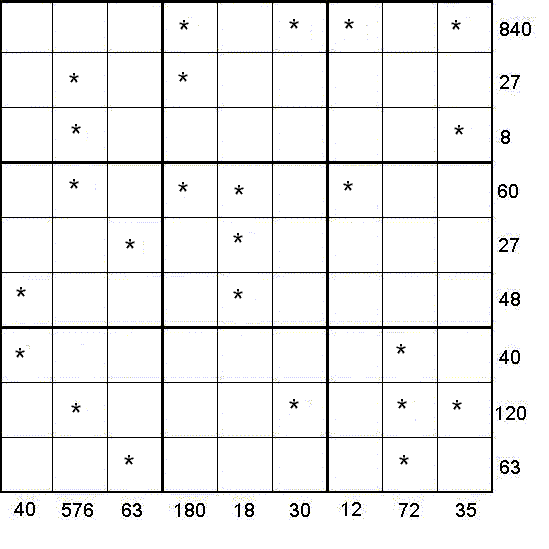#### You may also likeMake a set of numbers that use all the digits from 1 to 9, once and once only. Add them up. The result is divisible by 9. Add each of the digits in the new number. What is their sum? Now try some other possibilities for yourself!### N000ughty Thoughts

How many noughts are at the end of these giant numbers?### DOTS Division

Take any pair of two digit numbers x=ab and y=cd where, without loss of generality, ab > cd . Form two 4 digit numbers r=abcd and s=cdab and calculate: {r^2 - s^2} /{x^2 - y^2}.

# Star Product Sudoku

##### Age 11 to 16 Challenge Level:

By Henry KwokRules of Multiplication Equation Sudoku
Like the standard sudoku, this sudoku variant has two basic rules:
1. Each column, each row and each box ($3\times3$ subgrid) must have the numbers $1$ through $9$.
2. No column, row or box can have two squares with the same number.
The puzzle can be solved by finding the values of the unknown digits (all indicated by asterisks) in the squares of the $9\times9$ grid. At the bottom and right side of the $9\times9$ grid are numbers, each of which is the product of a column or row of unknown digits marked by asterisks. Altogether a set of 18 equations can be formed from the columns and rows of unknown digits and constants.

For example, in the first and second columns beginning from the left of the $9\times9$ grid, the following equations can be formed:
$*\times* = 40$
and
$*\times*\times*\times* = 576$.

In the fourth and fifth rows beginning from the top of the $9\times9$ grid, the following equations can be formed:
$*\times*\times*\times* = 60$
and
$*\times* = 27$.

After solving all the equations, the puzzle is solved by the usual sudoku technique and strategy.